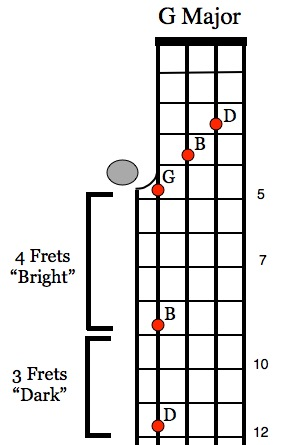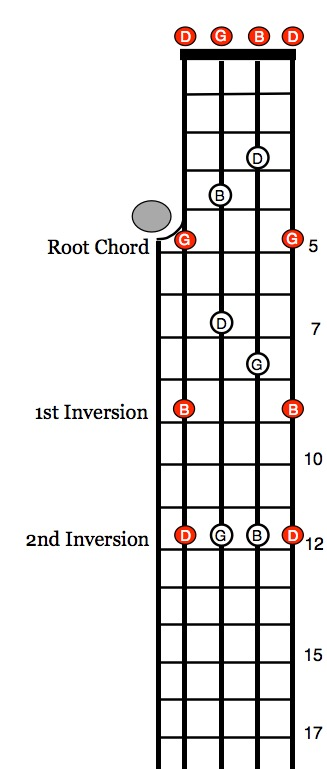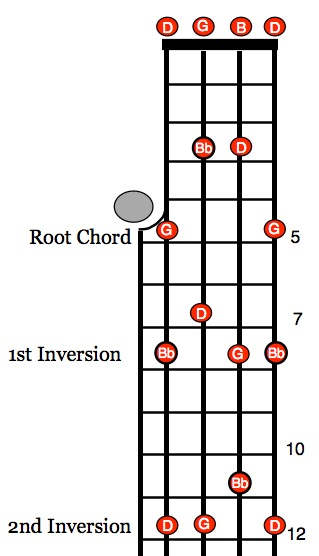# Chords Declassified 2

Chords Declassified!

Banjo Chord Theory Part 2

IntervalsIntervals have all kinds of interesting names. There are Major, Minor, Augmented, Diminished and Perfect intervals. There are also 2nds, 3rds, 4ths, 5ths, 6ths, 7ths and 8ths (Octave) intervals.

The Major Chord IntervalsAn interval is the distance between two notes measured from the lowest note. To demonstrate interval distance, we’ll use the length of the fourth string to construct the notes of a G major chord G-B-D.

Starting at G on the 5th fret, we count up four frets to get a B note at the 9th fret. From that B note, we count up three more frets to the D at the 12th fret.

The illustration shows how the G, B and D notes found along the length of the fourth string translate over to the fourth, third and second strings forming a G major chord form at the fifth fret. A four fret distance is called “bright” or Major Third Interval. On top of this is a Minor Third Interval or :dark.”

A four fret interval plus a three fret interval built on top forms a G Major Chord Triad.

4+3 frets = G Major

The Major ChordRearranging the notes of a G chord triad does not change the basic sound. Using the same three G-B-D notes shown in red along the fourth string, here are the Root, 1st and 2nd inversions of a G triad chord. Here are the three standard G major chord forms up the neck in 3 positions. The chord forms include the octave note on the first string.

Root G Major Chord = G – B – D – (G)

1st Inversion = B – D – G – (B)

2nd Inversion = D – G – B – (D)

The Minor ChordTo make a Minor chord we simply reverse the two intervals. This produces a G Minor chord.

3+4 frets = G Minor Root Chord

Here are some basic chords that are made up of 4 and 3 fret interval distances:

Major = 4+3

Minor = 3+4

Diminished = 3+3

Augmented = 4+4

Dominant = 4+3+3